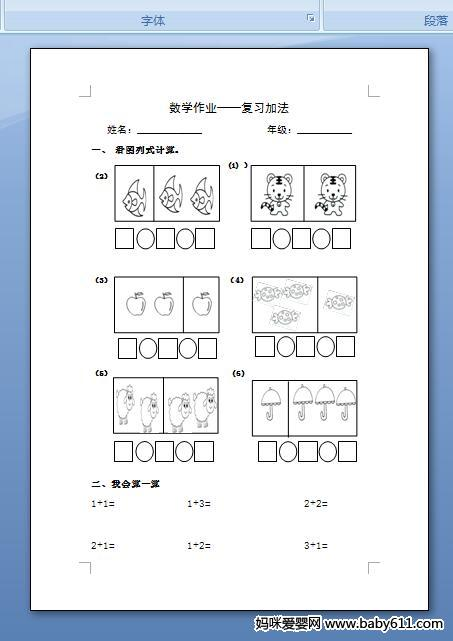# 幼儿园数学：4以内的加法练习题

数学作业--复习加法姓名：_____________             年级：___________一、看图列式计算。

(1)）（2）（3）（4）（5）     (6)二、我会算一算1+1=              1+3=                  2+2=2+1=              1+2=                  3+1=• 幼儿园试卷分类
• 将此试卷分享到：
• 数学试卷最新更新